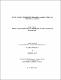## On the number of representations by quadratic forms and triangular numbers2021
##### Authors
Totani, Yash
University of Lethbridge. Faculty of Arts and Science
##### Publisher
Lethbridge, Alta. : University of Lethbridge, Dept. of Mathematics and Computer Science
##### Abstract
In this thesis, we study the problem of representing integers by quadratic forms. The formulas for the number of representations are obtained as a sum of an Eisenstein part and a cusp part. We begin by solving the representation problem for binary quadratic forms of discriminant -D<0 where the number field Q(√−D) has class number 3. We obtain formulas for the number of representations of an integer as a sum of k triangular numbers, denoted by δk(n), for even values of k. As special cases, for k=14,16 and 18, new formulas are provided in which the cusp part is given as a linear combination of certain eta products. At the end, for even values of k, we study the first and the second moments of δk(n) and prove an analogue of the Wagon's conjecture for the second moment of δk(n).
##### Keywords
number theory , quadratic forms , triangular numbers , Number theory , Forms, Quadratic , Dissertations, Academic